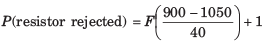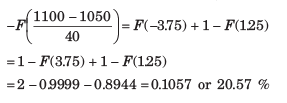Courses

# Random Variables - MCQ Test

## 20 Questions MCQ Test GATE ECE (Electronics) 2022 Mock Test Series | Random Variables - MCQ Test

Description
This mock test of Random Variables - MCQ Test for Electronics and Communication Engineering (ECE) helps you for every Electronics and Communication Engineering (ECE) entrance exam. This contains 20 Multiple Choice Questions for Electronics and Communication Engineering (ECE) Random Variables - MCQ Test (mcq) to study with solutions a complete question bank. The solved questions answers in this Random Variables - MCQ Test quiz give you a good mix of easy questions and tough questions. Electronics and Communication Engineering (ECE) students definitely take this Random Variables - MCQ Test exercise for a better result in the exam. You can find other Random Variables - MCQ Test extra questions, long questions & short questions for Electronics and Communication Engineering (ECE) on EduRev as well by searching above.
QUESTION: 1

### The number of cars arriving at ICICI bank drive-in window during 10-min period is Poisson random variable X with b = 2. Que : The probability that more than 3 cars will arrive during any 10 min period is

Solution: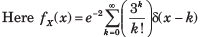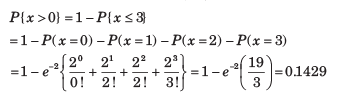QUESTION: 2

### The number of cars arriving at ICICI bank drive-in window during 10-min period is Poisson random variable X with b = 2. Que: The probability that no car will arrive is

Solution: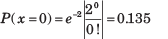QUESTION: 3

### The power reflected from an aircraft of complicated shape that is received by a radar can be described by an exponential random variable W. The density of W is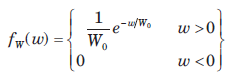where W0 is the average amount of received power. The probability that the received power is larger than the power received on the average is

Solution: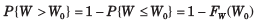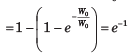QUESTION: 4

Delhi averages three murder per week and their occurrences follow a poission distribution

Que: The probability that there will be five or more murder in a given week is

Solution: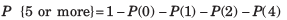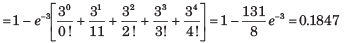QUESTION: 5

Delhi averages three murder per week and their occurrences follow a poission distribution

Que: On the average, how many weeks a year can Delhi expect to have no murders ?

Solution:

average number of week, per year with no murder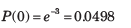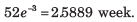QUESTION: 6

Delhi averages three murder per week and their occurrences follow a poission distribution.

Que:How many weeds per year (average) can the Delhi expect the number of murders per week to equal orexceed the average number per week ?

Solution: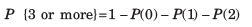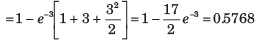Average number of weeks per year that number of murder exceeds the average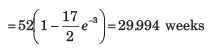QUESTION: 7

A discrete random variable X has possible values x i = i2  i =1, 2, 3, 4 which occur with probabilities 0.4, 0.25, 0.15, 0.1,. The mean value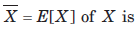Solution: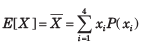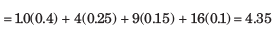QUESTION: 8

The random variable X is defined by the density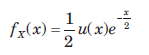The expected value of g(X)  = X3 is

Solution: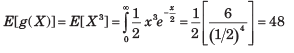QUESTION: 9

The random variables X and Y have variances 0.2 and 0.5 respectively. Let Z= 5X-2Y. The variance of Z is?

Solution:

Var(X) = 0.2, Var(Y) = 0.5
Z = 5X – 2Y
Var(Z) = Var(5X-2Y)
= Var(5X) + Var(2Y)
= 25Var(X) + 4Var(Y)
Var(Z) = 7.

QUESTION: 10

The variance of random variable X is

Solution:

Solution=

Variance of X is σx2=E[X2]- μx2

E(X2)=-∞ x2fx(x)dx=01  x2(x)3(1-x)2dx=1/10

σx2 =(1/10)-(1/4)2=3/80

hence option B would be the correct answer.

QUESTION: 11

A Random variable X is uniformly distributed on the interval (-5, 15). Another random variableY =  e -X/5 is formed. The value of E[Y ] is

Solution: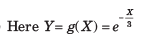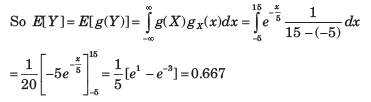QUESTION: 12

A joint sample space for two random variable X and Y has four elements (1, 1), (2, 2), (3, 3) and (4, 4). Probabilities of these elements are 0.1, 0.35, 0.05 and 0.5 respectively.

Que: The probability of the event {X ≤ 2.5, Y ≤ 6} is

Solution: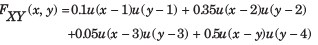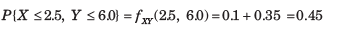QUESTION: 13

A joint sample space for two random variable X and Y has four elements (1, 1), (2, 2), (3, 3) and (4, 4). Probabilities of these elements are 0.1, 0.35, 0.05 and 0.5 respectively.

Que: The probability of the event {X ≤ 3} is

Solution: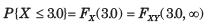= 0.1+ 0.35 + 0.05 = 0.5

QUESTION: 14

The statistically independent random variable X and Y have mean values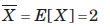.and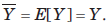They have second moments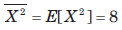and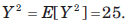Consider a random variable W = 3X - Y.

Que: The mean value E [W] is

Solution: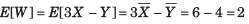QUESTION: 15

The statistically independent random variable X and Y have mean values.andThey have second momentsandConsider a random variable W = 3X - Y.

Que: The second moment of W is

Solution: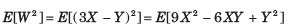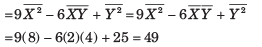QUESTION: 16

The statistically independent random variable X and Y have mean values.andThey have second momentsandConsider a random variable W = 3X - Y.

Que: The variance of the random variable is

Solution: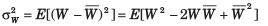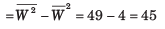QUESTION: 17

Two random variable X and Y have the density function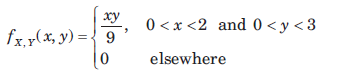The X and Y are

Solution: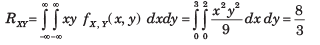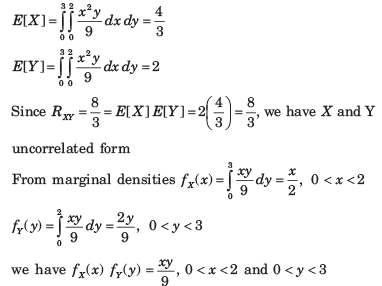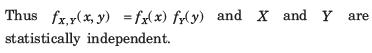QUESTION: 18

The value of σx2 , σy2 , RXY and ρ are respectively

Solution: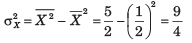QUESTION: 19

The mean value of the random variable

W = (X + 3Y)2  + 2X + 3  is

Solution: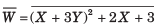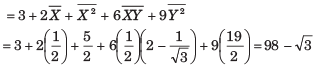QUESTION: 20

If machine is not properly adjusted, the product resistance change to the case where ax = 1050Ω . Now the rejected fraction is

Solution: